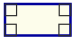# Parallelogram

A Parallelogram is a flat shape with opposite sides parallel and equal in length.| and || show equal sidesOpposite sides are parallelOpposite sides are equal in lengthOpposite angles are equal (angles "a" are the same, and angles "b" are the same)Angles "a" and "b" add up to 180°, so they are supplementary angles.

Play with a Parallelogram:

NOTE: Squares, Rectangles and Rhombuses are all Parallelograms!

### Example:A parallelogram where all angles are right angles is a rectangle!

## Area of a ParallelogramThe Area is the base times the height: Area = b × h (h is at right angles to b)

### Example: A parallelogram has a base of 6 m and is 3 m high, what is its Area?

Area = 6 m × 3 m = 18 m2

## Perimeter of a Parallelogram

The Perimeter is the distance around the edges.The Perimeter is 2 times the (base + side length): Perimeter = 2(b+s)

### Example: A parallelogram has a base of 12 cm and a side length of 6 cm, what is its Perimeter?

Perimeter = 2 × (12 cm + 6 cm) = 2 × 18 cm = 36 cm

## Diagonals of a ParallelogramThe diagonals of a parallelogram bisect each other.

In other words the diagonals intersect each other at the half-way point.